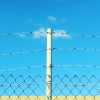{meta: 原调: 1=A} {meta: 选调: 1=G} {meta: Capo: 2} {meta: 参考速度: 63} {meta: 拍子: 4/4} {comment: 备注} {comment: 一、为了方便掌握歌词时值，和弦落拍处会尽量对齐。} {comment: 二、本曲有一小节两歌和弦的情况，为了方便阅读，统一每小节标注两个和弦。} {comment: Verse 1} [G]小时候　　我[G]总　会这样　[C]牵着你　的[C]手 [Am7]只是盼望　　[D]能够在你　　[G]的身边　守[G]候 {comment: Verse 2} [G]为了保　　护[G]你　不小心　[C]割破手　指[C]头 这个[Am7]小伤却让[D]你　泪流心　[G]痛　　　　[G] {comment: Pre-Chorus} 长大后[B]我们越来[B]越远　分隔[Em]地球的　两[Em]边 何[C]时才能　够[G]见面　　　熟[Am7]悉微笑　的[D]脸 [D]　[D] {comment: Chorus 1} {start_of_chorus} [G]　回忆起[G]我们小时[Em]候　闭上眼[Em]睛就能够感[C]受 在我们[G/B]心中慢慢[Am7]流动的　温[D]柔 [G]　离开了[G]我们小时[Em]候　现在你[Em]会　不会想[C]我 也许你[G/B]找到一个[Am7]人为你　守[D]候　我了[G]了　　　[G] {end_of_chorus} [G]　 {comment: Verse 1} [G]小时候　　我[G]总　会这样　[C]牵着你　的[C]手 [Am7]只是盼望　　[D]能够在你　　[G]的身边　守[G]候 {comment: Verse 2} [G]为了保　　护[G]你　不小心　[C]割破手　指[C]头 这个[Am7]小伤却让[D]你　泪流心　[G]痛　　　　[G] {comment: Pre-Chorus} 长大后[B]我们越来[B]越远　分隔[Em]地球的　两[Em]边 何[C]时才能　够[G]见面　　　熟[Am7]悉微笑　的[D]脸 {comment: Chorus 2} {start_of_chorus} [G]　回忆起[G]我们小时[Em]候　闭上眼[Em]睛就能够感[C]受 在我们[G/B]心中慢慢[Am7]流动的　温[D]柔 [G]　离开了[G]我们小时[Em]候　现在你[Em]会　不会想[C]我 也许你[G/B]找到一个[Am7]人为你　守[D]候 我了[G]了　回忆起[G]我们小时[Em]候　闭上眼[Em]睛就能够感[C]受 站在窗[G/B]前跟你说[Am7]了晚安　就[D]走 [G]　离开了[G]我们小时[Em]候　现在的[Em]你不再　想[C]我 [G/B]这　个时[Am7]候　　　[D]我　了　　[G]了　　　　[G] {end_of_chorus} {title:我们小时候} {artist:Tank} {author:justin早安}justin早安